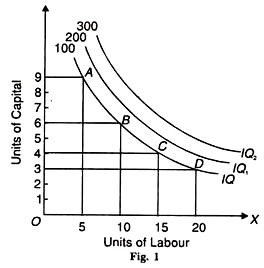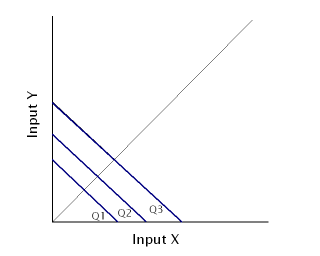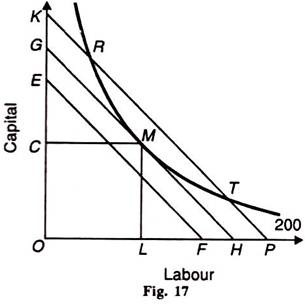# Producer’s Equilibrium through Isoquants

Economic production is the result of the output we produce by employing factors like land, labour, capital, and entrepreneurship. It is possible to determine the optimum amount of production possible considering different combinations of these inputs. Such a determination is called the producer’s equilibrium.

Producer’s Equilibrium

The value of all assets used for production is limited. Hence, the producer has to use such a combination of inputs as would provide him with maximum output and profits. This optimum level of production, also called producer’s equilibrium, is achieved when maximum output is derived from minimum costs.

In order to achieve this, producers first have to classify their resources into different combinations. Each combination would provide production in different quantities. The combination that provides the highest amount of produce at the least amount of costs is the optimum level of production.

In order to find out producer’s equilibrium, we first need to understand isoquant curves and iso-cost lines. These two concepts help us calculate optimum production.

Isoquant Curves

These lines represent various input combinations which produce the same levels of output. The producer can choose any of these combinations available to him because their outputs are always the same. Thus, we can also call them equal-product curves or production indifference curves.

Just like indifference curves, isoquants are also negatively-sloping and convex in shape. They never intersect with each other. When there are more curves than one, the curve on the right represents greater output and curves on the left show less output.

Consider the table below. It shows four combinations, i.e. A, B, C and D, which produce varying levels of output.

 Factor combinations Units of Labour Units of Capital A 5 9 B 10 6 C 15 4 D 20 3

Plotting these figures on a graph provides us with this curve (Figure 1):The X-axis shows units of labour, while the Y-axis represents units of capital. Points A, B, C and D are combinations of factors on which IQ is the level of output, i.e. 100 units. IQ1 and IQ2 represent greater potential output.

Isocost Lines

Isocost lines represent combinations of two factors that can be bought with different outlays. In other words, it shows how we can spend money on two different factors to produce maximum output. These lines are also called budget lines or budget constraint lines.

Let’s assume that a farmer has Rs. 1,000 to spend on labour costs and ploughs for farming. The cost of one such plough and wage per labourer is Rs. 100. Considering his total outlay of Rs. 1,000, he can spend that money in the following combinations:

 Ploughs 0 100 200 300 400 500 600 700 800 900 1000 Labour 1000 900 800 700 600 500 400 300 200 100 0

The farmer, in this case, can either spend the entire sum of Rs. 1,000 on just ploughs by buying 10 of them. Similarly, he can also spend it all on labour by employing 10 labourers. He can even purchase both, labour and ploughs using different combinations as shown above. The total outlay of Rs. 1,000 will remain the same. Hence, the isocost line will remain straight as shown below:The x-axis represents units of ploughs, and Y-axis would show units of labour. Output levels are shown by a straight line because they remain constant.

Production Equilibrium

Isoquant curves, as we learned above, show us input combinations that we can employ to produce certain levels of output. Furthermore, isocost lines help us determine combinations of two factors in which we can invest our outlays to produce output. A combination of these two graphs is what gives us the optimum production level, i.e. the producer’s equilibrium.

Using this equilibrium, the producer can determine different combinations to increase output. He can also use this information to find ways to cut costs using the same inputs and consequently generate more profit. We can find out the least expensive combinations of factors by superimposing isoquant curves on isoquant lines.

Plotting Producer’s Equilibrium

The graph below shows how we can use isoquant curve and isocost lines to determine optimum producer’s equilibrium.In the figure shown above, the isoquant curve represents targeted output, i.e. 200 units. Icocost lines EF, GH and KP show three different combinations in which we can utilize the total outlay of inputs, i.e. capital and labour.

The isoquant curve crosses all three isocost lines on points R, M and T. These points show how much costs we will incur in producing 200 units. All three combinations produce the same output of 200 units, but the least costly for the producer will be point M, where isocost line GH is tangent to the isoquant curve.

Points R and T also cross the isoquant curve and equally produce 200 units, but they will be more expensive because they are on the higher isocost line of KP. At point R the producer will spend more on capital, and labour will be more expensive on point T.

Thus, point M is the producer’s equilibrium. It will produce the same output of 200 units, but will a more profitable combination as it will cost less. The producer must, therefore, spend OC amount on capital and OL amount on labour.#### intactone

View all posts by intactone →
error: Content is protected !!